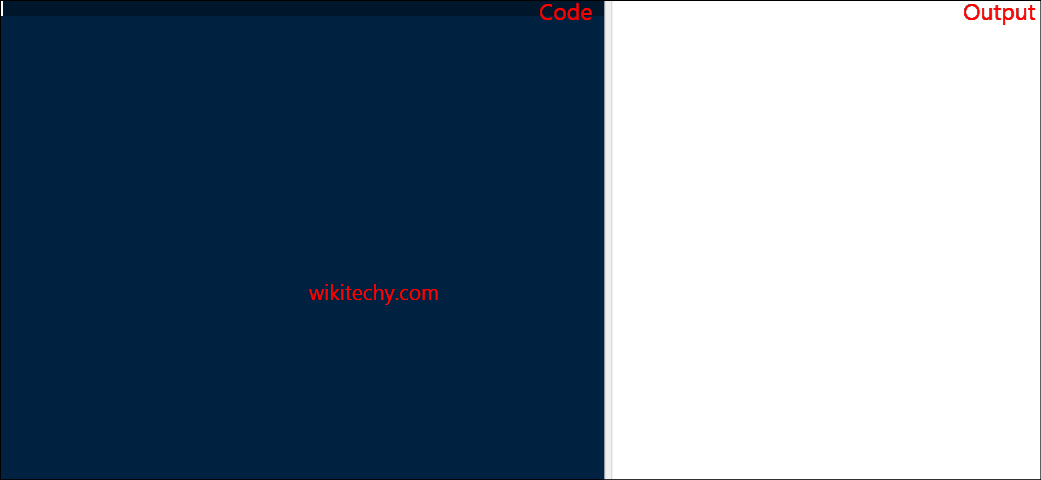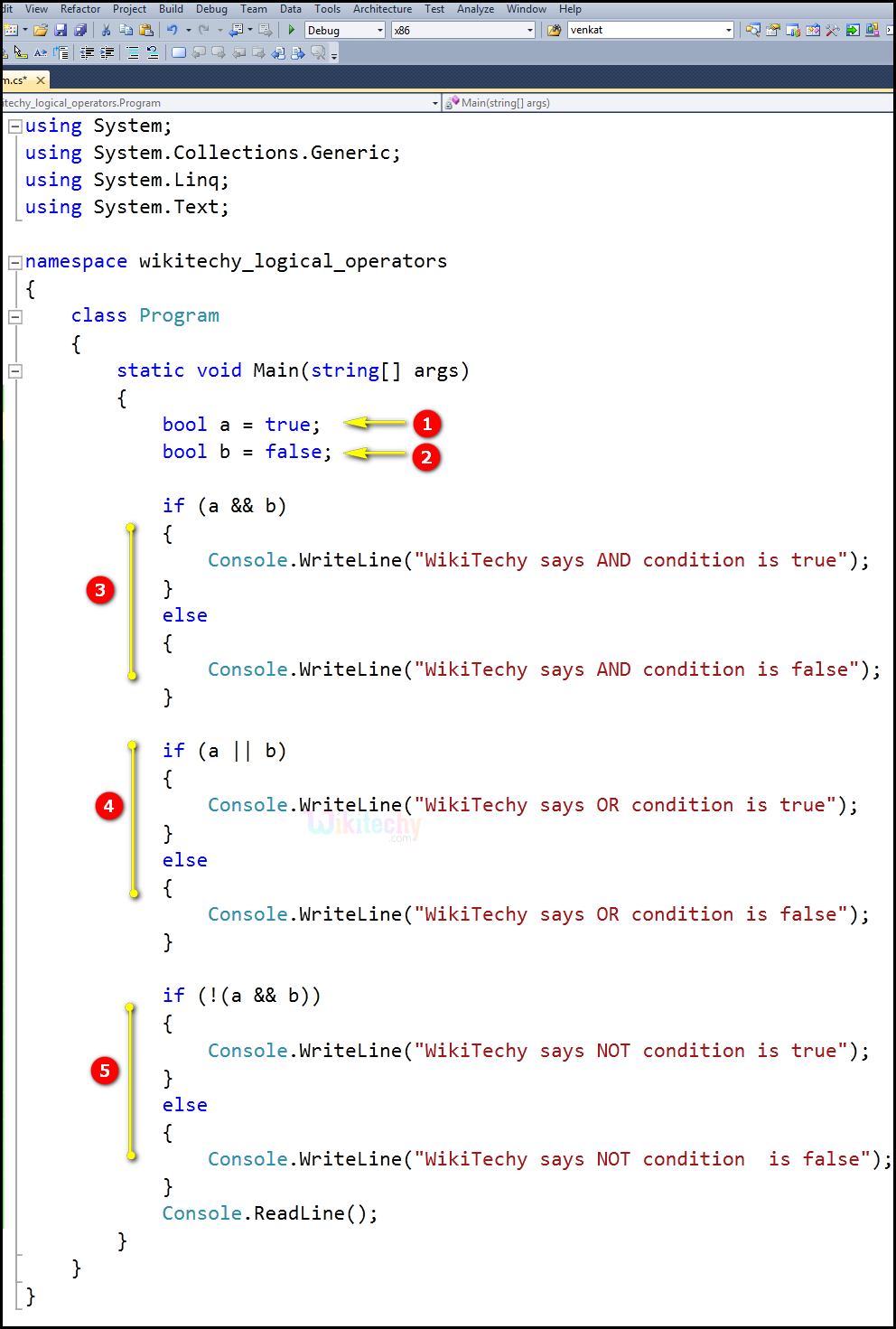# C# Logical Operators - c# - c# tutorial - c# netC# Logical Operators

## What is logical operators in C# ?

• The C# Logical Operator also evaluates the values and returns true or false as output.
• These based on true-false the program performs dynamically at a run time.
• The logical operator is generally used with C# programming.
S.no Operators Name Example Description
1 && logical AND (x>5) && (y<5) It returns true when both conditions are true
2 || logical OR (x>=10) || (y>=10) It returns true when at least one of the condition is true
3 ! logical NOT !((x>5)&&(y<5)) It reverses the state of the operand "((x>5) && (y<5))"

If "((x>5) && (y<5))" is true, logical NOT operator makes it false.Logical Operator

## Code Explanation:1. bool a = true bool a stores true condition. Bool variables can be assigned values based on expressions. Many expressions evaluate to a Boolean value. Represented in one byte, the bool type represents truth.
2. bool b = false bool b stores false condition. Bool variables can be assigned values based on expressions. Many expressions evaluate to a Boolean value. Represented in one byte, the bool type represents false.
3. In this statement we are using the logical operator AND (a && b) for the condition of checking true && false, which will be not satisfied because a=true and b=false, so the output go to the else statement "false", which will be printed in the statement "WikiTechy says AND condition is false".
4. In this statement we are using the logical operator OR (a || b) for the condition of checking true || false, which will be satisfied because a=true and b=false, so the output set as "true", which will be printed in the statement "WikiTechy says OR condition is true".
5. In this statement we are using the logical operator NOT (! (a && b)) for the condition of checking (not (true || false)), which will be satisfied because a=true and b=false, so the output set as "true", which will be printed in the statement "WikiTechy says NOT condition is true".

## Sample C# examples - Output :1. Here in this output, we have shown the "WikiTechy says AND condition is false", which specifies to else statement. Because the AND (a && b) logical operator condition is not satisfied.
2. Here in this output, we have shown the "WikiTechy says OR condition is true", which specifies to OR (a || b) logical operator condition is not satisfied.
3. In this output, we have shown the "WikiTechy says NOT condition is true", which specifies to NOT (! (a && b) logical operator condition is not satisfied.